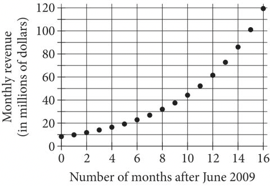# The Difference Between SAT and ACT Math

Sep 06, 2022• Structure and Score
• Time
• Topics and Question Types
• SAT Math Topics
• ACT Math Topics
• Conclusion

# Structure and Score

Two obvious differences between SAT and ACT math is that the SAT has two math sections, a calculator and a no calculator section, while the ACT has one and the SAT has free response questions while all the questions on the ACT are multiple choice. These differences are important. Looking at this since both tests have 4 sections, you may think that 50% of your SAT score is from math and only 25% of your ACT score is based on math. Don’t forget that the Science section on the ACT is reading charts and graphs, a math skill. You will want to look at other differences between SAT and ACT math before you make your decision which test is right for you.

# Time

Another apparent difference between SAT and ACT math is time. The ACT has 60 questions and allows 60 minutes. Pretty easy math there, one minute per question. The SAT has 38 questions on its Math Calculator section and allows 55 minutes, one minute 26 seconds per question. On the No Calculator section there are 20 questions to be completed in 25 minutes, 1 minute 15 seconds per question. Don’t base your decision  on time allotted alone. There is a reason the SAT allows more time per question and it is NOT to make the test easier.

# Topics and Question Types

The SAT tests fewer concepts but requires students to understand them in depth. The ACT tests a much wider range of concepts but the questions are much more straightforward.

## SAT Math Topics

• linear equations and inequalities (solving, graphing, interpreting)
• exponential and quadratic equations (solving, graphing, interpreting)
• systems of equations (solving, number of solutions, word problems)
• radicals and rational exponents (solving, operations)
• center, spread and shape of graphs (data analysis)
• scatter plots

## ACT Math Topics

• ### Geometry

• area of triangles, special right triangles, Pythagorean Theorem, isosceles, equilateral, similar, external angles)
• circles, area and circumference, sectors, angles and arcs, tangent, chords, radius and diameter
• quadrilaterals, area and perimeter, diagonals, internal angles
• polygons, interior angles, area of embedded shapes
• ellipses
• ### Statistics and Probability

• probability
• percent change
• patterns and sequences
• ### Functions

• solving, graphing compositions
• ### Algebra 1 and 2

• linear equations, solving, graphing, slope intercept form, slope
• parallel lines, perpendicular lines, midpoint, distance
• exponential decay and growth
• systems of equations
• range and domain
• matrices
• unit circle
• logarithms
• imaginary and complex numbers
• combinations and permutations
• ### Number and Quantity

• adding, subtracting, multiplying and dividing fractions
• number properties
• absolute value
• scientific notation
• ### Pre-Algebra

• mean, median and mode
• volume and surface area of 3 D shapes
• area and perimeter of shapes
• ratios and inequalities
• percentages

As you can see a substantial difference between SAT and ACT math is that the SAT has very little geometry, between 5 and 10 questions out of 58 total questions (10 - 15%). The ACT is approximately 30% geometry questions. SAT also provides basic geometric formulas on the first page of the test. The ACT occasionally provides information in the question.

Another important difference between SAT and ACT math is that the SAT tests concepts as well as calculations. Students will be asked the significance of a particular value or variable in an equation. The ACT is much more like questions on a school math test. Here is the information, now solve the problem.

### Example SAT Math problemThe graph shown models the monthly marketing revenue, in millions of dollars, for a particular company from June 2009 to October 2010. According to the model, which of the following is closest to the company’s monthly revenue, in millions of dollars, for June 2009?

1. 8.5
2. 22.5
3. 62
4. 100

􏰇􏰚􏰰􏰛􏱂􏰵􏰾􏰗􏰾􏰽􏱅􏰰􏱃􏰧􏰗􏰥􏰽􏱀

### 􏰝􏰄􏰈􏰇􏰋􏰫􏰈􏰒Example ACT Math Problems

Given functions f(x) = 5x + 1 and g(x) = x2 - 2 􏰎􏰋􏰒􏰈􏰂􏰊 􏰌􏰓􏰒􏰃􏰖􏰋􏰉􏰒 􏰇 􏰔􏰉􏰐􏰈􏰎what is the value of f(g(-3))?

1.  -198
2. -54
3. -39
4. 36
5.  194􏰖􏰄􏰈 􏰂􏰒􏰒􏰓􏰂􏰎 􏰅􏰈􏰊􏰃􏰈􏰒􏰖􏰂􏰇􏰈􏰋􏰒􏰃􏰊􏰈􏰂􏰆􏰈􏰋􏰒􏰖􏰄􏰈􏰅􏰉􏰅􏰓􏰎􏰂􏰖􏰋􏰉􏰒􏰉􏰌
􏰮􏰒􏰐􏰋􏰂 􏰰 􏰑􏰈􏰂􏰊􏰆 􏰂􏰌􏰖􏰈􏰊 􏰥􏰦􏰦􏰾􏰗 􏰬􏰄􏰂􏰖 􏰋􏰆 􏰖􏰄􏰈 􏰍􏰈􏰆􏰖 􏰋􏰒􏰖􏰈􏰊􏰅􏰊􏰈􏰖􏰂􏰖􏰋􏰉􏰒 􏰉􏰌 􏰇􏰚􏰧􏰾􏰛􏱂􏰥􏰗􏰽􏱆􏱀 􏰋􏰒 􏰖􏰄􏰋􏰆 􏰃􏰉􏰒􏰖􏰈􏰰􏰖􏰴

## Conclusion

The difference between the SAT and ACT math sections are the greater than any other section and can dramatically impact a student’s score. It is important to familiarize yourself with those differences when deciding which test is best for you. Here are some questions you may want to ask yourself while deciding

• Am I good at remembering facts and formulas?  (ACT)
• Do I work quickly and accurately? (ACT)
• Do I understand mathematical concepts as well as operations? (SAT)
• Am I good at Geometry? (ACT)
• Do word problems intimidate me? (ACT)
• Am I good at graphing? (SAT)
• Do I depend on my calculator? (ACT)

I would also recommend taking the math portion of each test. It will take a total of 2 hours and 30 minutes, a wise time investment for such an important test.